# Modifying Geometrical TolerancesThis task shows you how to modify a geometrical tolerance.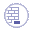Open the Brackets_views03.CATDrawing document. Create a geometrical tolerance.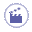1. Double-click the geometrical tolerance you want to modify.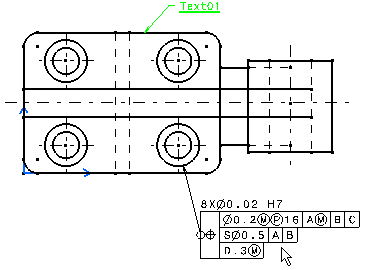The Geometrical Tolerance dialog box is displayed, with the existing values pre-entered.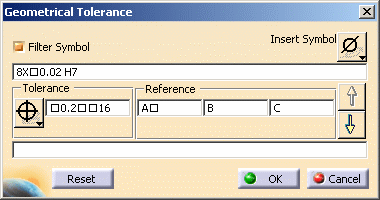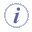You can reset the current style values in the Geometrical Tolerance dialog box at any time using the Reset button.

2. Modify the values as desired, as explained in Creating a Geometrical Tolerance.

3. Click OK.

4. Click in the free space to validate the geometrical dimension modification.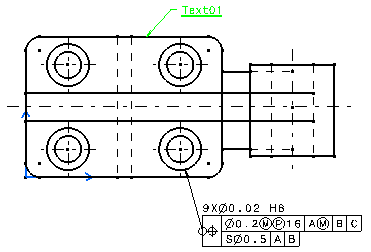5. Create a dimension, then create a geometrical tolerance on it.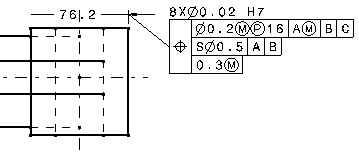6. Click on the extension line and move the dimension.
You can see that the geometrical tolerance follows the dimension.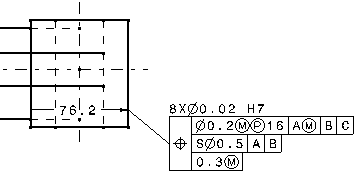7. Click on the geometrical tolerance and move it.
You can see that it has not impact on the position of the dimension.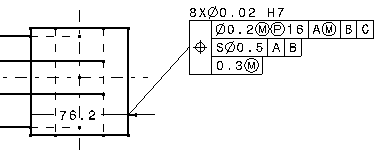The behavior is the same if a geometrical tolerance is created on the dimension value.

### Associativity between the dimension and the geometrical tolerance

1. Create a dimension.

2. Create a geometrical tolerance on it, selecting the dimension line.

3. Move the dimension.
You will note that the positioning and the length of the tolerance leader remain constant when moving the dimension, whatever the dimension type.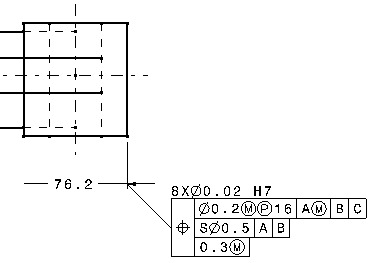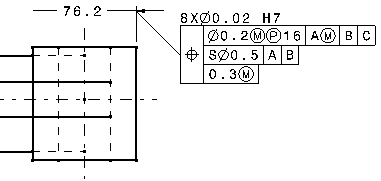5. Create a dimension.

6. Create a geometrical tolerance on it, selecting the extension line.

7. Move the dimension.
You will note the length of the tolerance leader is recomputed as long as the dimension is moved.
This is due to the fact that the ratio between the lengths of the leader's projection and the extension line's projection remain constant.

The positioning of the leader is also recomputed so that the distance between the anchor point of the tolerance and the extension line remain constant.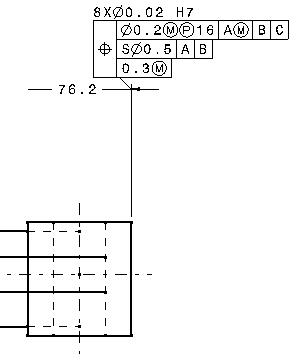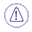When a tolerance is created on an angle dimension, the positioning and the length of the leader remain constant if the dimension is moved.